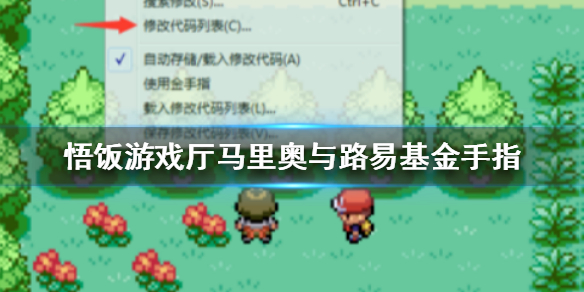# 悟饭游戏厅马里奥与路易基rpg金手指代码大全1、基础代码

2、使用

3、物品金手指

(该金手指是偶在网上找的EC码翻印过来的，经本人测试完全可用。此为VBA码...希望大家能喜欢！)

E2=蘑菇

E3=超级蘑菇

E4=终极蘑菇

E5=MAX蘑菇

E6=坚果

E7=超级坚果

E8=终极坚果

E9=MAX坚果

F1=鲜草药

F2=红辣椒

F3=绿辣椒

F4=哈哈纯咖啡

F5=呵呵纯咖啡

F6=啦啦纯咖啡

F7=嘿嘿纯咖啡

F8=呵呵浓咖啡

F9=啦啦浓咖啡

F0=黄金蘑菇